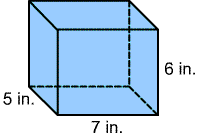Skill 23C
Area
 What is the formula to find the volume of the rectangular prism?a. V = l x w b. V = l x w x h c. V = h x l d. not sure